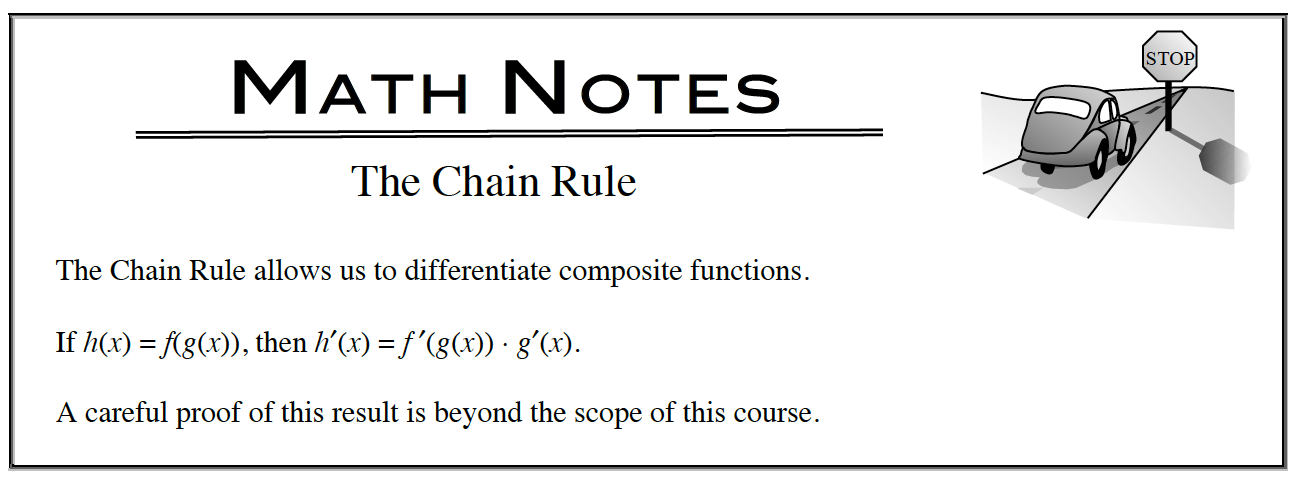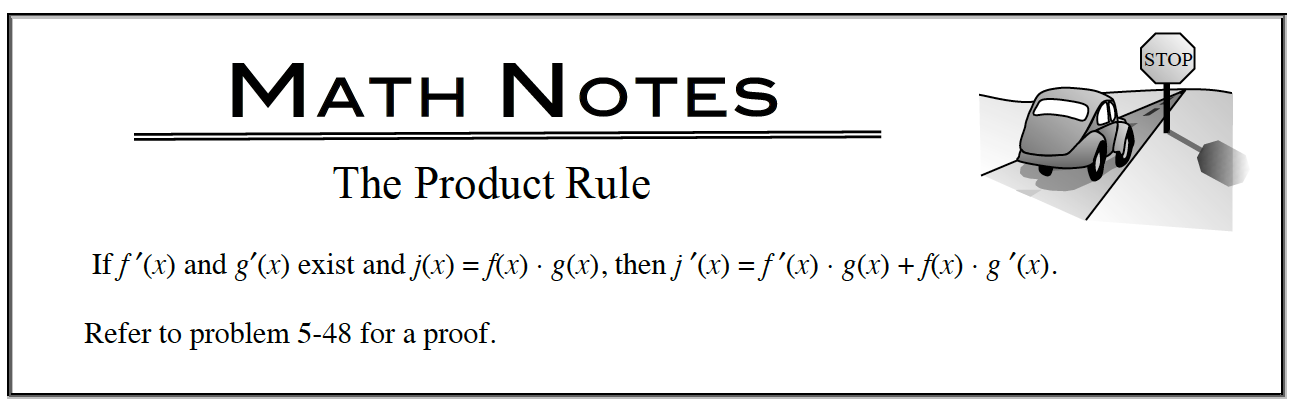### Home > APCALC > Chapter 6 > Lesson 6.1.2 > Problem6-24

6-24.

First, decide if differentiating the following expressions requires the Product Rule, the Quotient Rule, the Chain Rule, or a combination of these rules. Then, evaluate the following derivatives. Homework Help ✎

1. $\frac { d } { d x }$$[ f ( t ^ { 2 } ) ]$2. $\frac { d } { d x }$$[ x \cdot h ( x ) ]$3. $\frac { d } { d x }$$[ t \cdot h ( t ^ { 2 } ) ]$